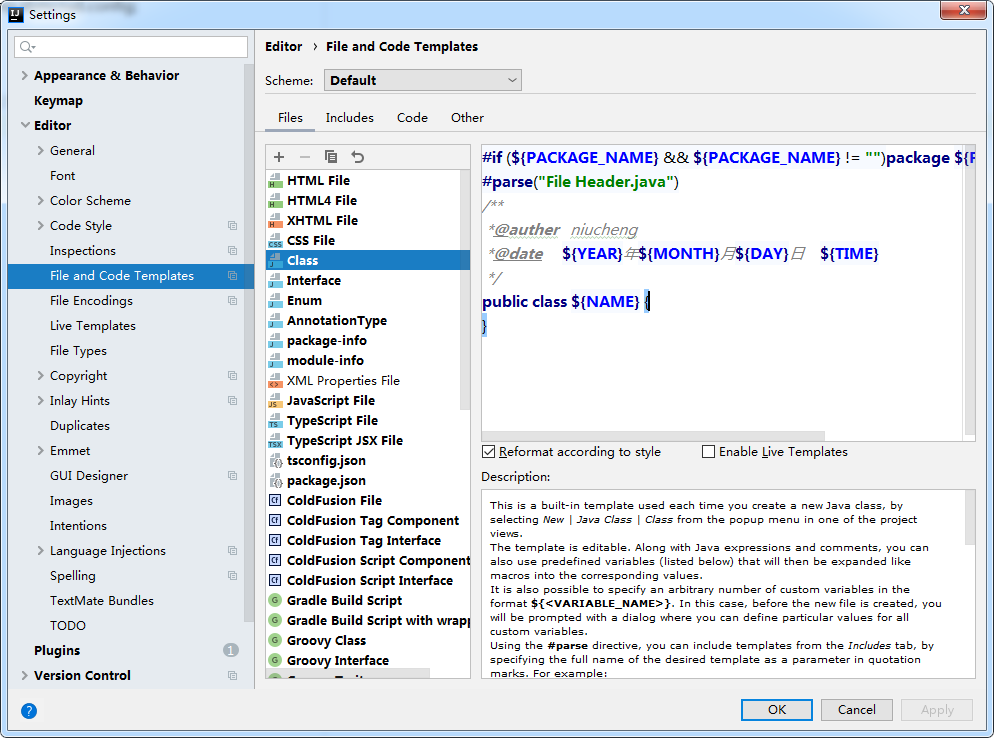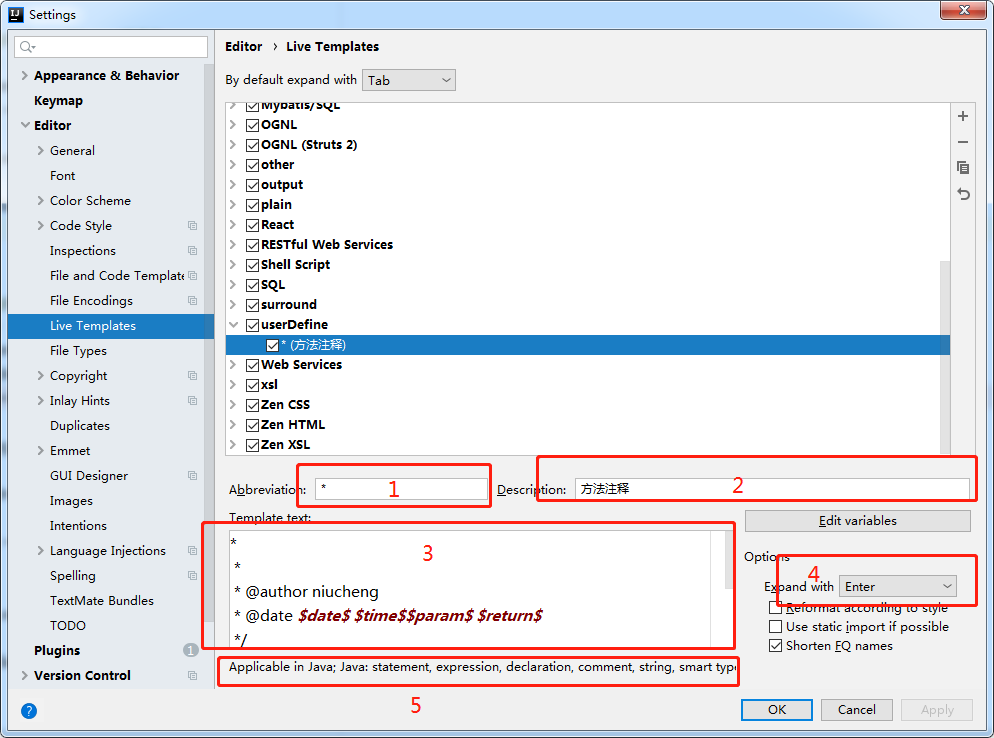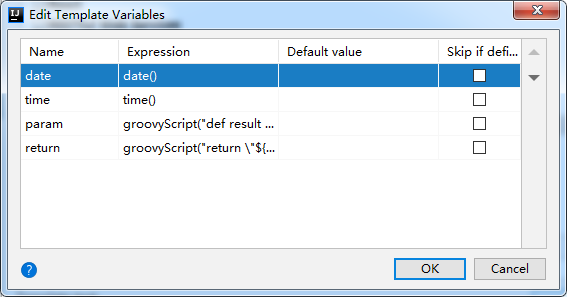# 我的idea注释配置

• 时间:
• 浏览:``````/**
*@auther   niucheng
*@date     \${YEAR}年\${MONTH}月\${DAY}日    \${TIME}
*/````````````*
*
* @author niucheng
* @date \$date\$ \$time\$\$param\$ \$return\$
*/```````ate` 和 `time` 的 `Expression` 使用的是 IDEA 内置的函数，直接使用下拉框选择就可以了，而 `param` 这个参数 IDEA 默认的实现很差，因此我们需要手动实现，

param：

``````groovyScript("def result = '';def params = \"\${_1}\".replaceAll('[\\\\[|\\\\]|\\\\s]', '').split(',').toList(); for(i = 0; i < params.size(); i++) {if(params[i] != '')result+='* @param ' + params[i] + ((i < params.size() - 1) ? '\\r\\n ' : '')}; return result == '' ? null : '\\r\\n ' + result", methodParameters())
``````

return

``````groovyScript("return \"\${_1}\" == 'void' ? null : '\\r\\n * @return ' + \"\${_1}\"", methodReturnType())
``````